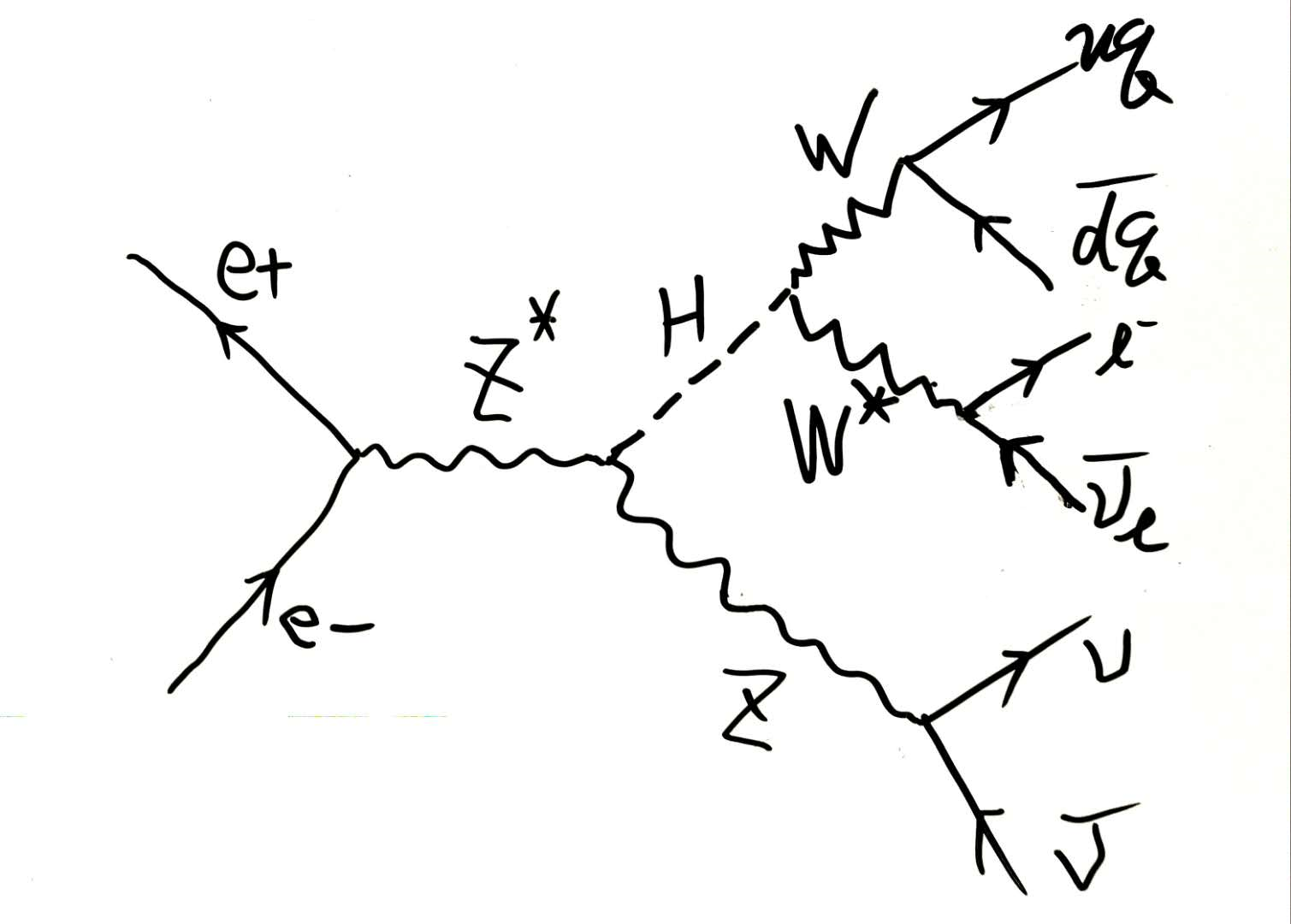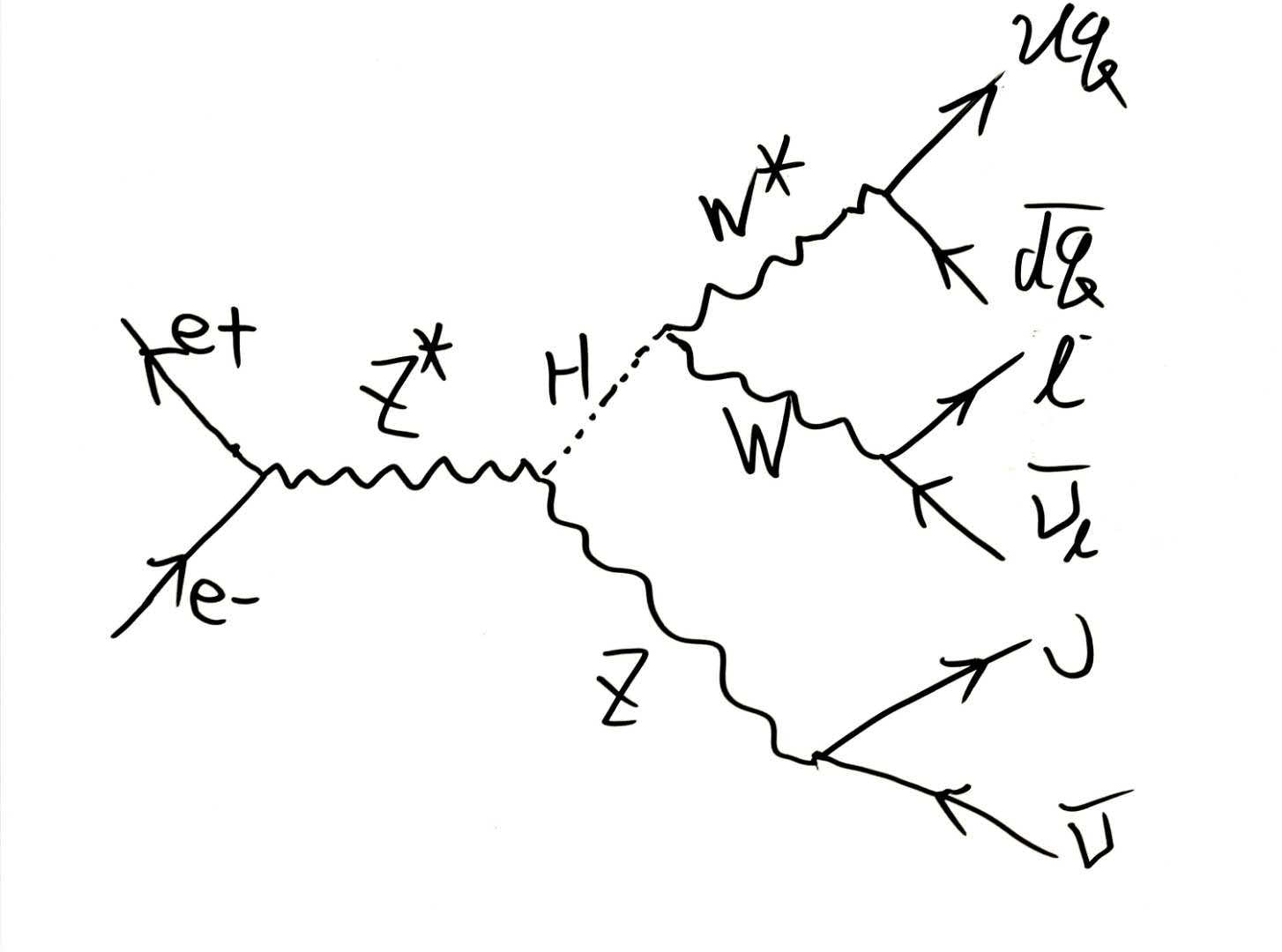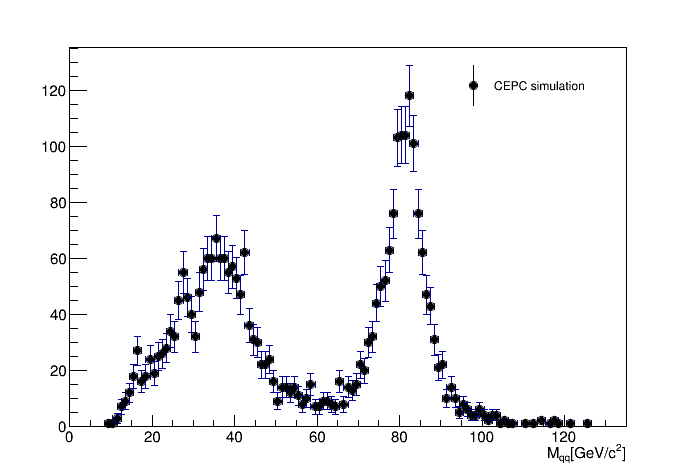# vvH, H->di W Analysis

• You may find the project at http://cepcgit.ihep.ac.cn/lihawl/vvHWW. The website is open to register. When you can access the project, use git clone git@cepcgit.ihep.ac.cn:lihawl/vvHWW.git to have a local copy of this project.

## Introduction

In the process of vvH, H->WW, WW->lvqq, the invariant mass of di-jet ($qq$) is just the module of 4-momentum of $W$. One $W$ of di-W boson is real, and the orther is virtual, as shown in the figures below respectively.It is expected there are one sharp peak at $W$ mass and a bump at lower energy than the W mass in the invariant mass spectrum of di-jet.

## Event Selection

• The input sample is the vvH, H->WW, WW inclusively decay. We firstly select the final state $\mu vqq$ out using the Monte Carlo truth information.
• Then we need to find out the muon which comes from $W$. This muon is just identified as the $\mu^+$ or $\mu^-$ with maximum energy.
• Finally, the invariant mass of $qq$ is calculated by $M_{qq} = \sqrt{(P_{\rm visible} - P_{\mu})^2}.$ Jet clustering is not needed.

## Result

The invariant mass of di-jet from W